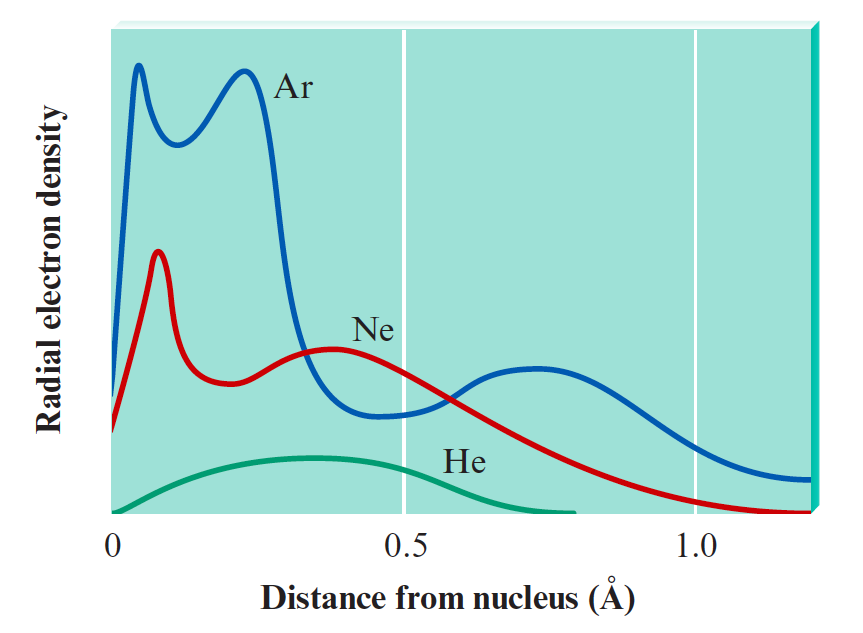Chemistry Practice Problems Quantum Numbers Practice Problems Solution: Total radial probability distributions for the hel...

⚠️Our tutors found the solution shown to be helpful for the problem you're searching for. We don't have the exact solution yet.

# Solution: Total radial probability distributions for the helium, neon, and argon atoms are shown in the following graph. How can one interpret the shapes of these curves in terms of electron configurations, quantum numbers, and nuclear charges?

###### Problem

Total radial probability distributions for the helium, neon, and argon atoms are shown in the following graph. How can one interpret the shapes of these curves in terms of electron configurations, quantum numbers, and nuclear charges?Quantum Numbers

Quantum Numbers

#### Q. What are the possible values of ml for each of the following values of l? Part C2Express your answers as integers in ascending order separated by comm...

Solved • Wed Sep 19 2018 14:03:08 GMT-0400 (EDT)

Quantum Numbers

#### Q. What are the possible values of ml for each of the following values of l? Part A0Express your answers as integers in ascending order separated by comm...

Solved • Wed Sep 19 2018 13:59:40 GMT-0400 (EDT)

Quantum Numbers

#### Q. How many electrons in an atom could have these sets of quantum numbers? n=3 n=4, l=2 n=6, l=2, ml = -1

Solved • Tue Aug 21 2018 17:21:42 GMT-0400 (EDT)

Quantum Numbers

#### Q. What quantum numbers specify these subshells?

Solved • Tue Aug 21 2018 17:18:36 GMT-0400 (EDT)